%run src/init_notebooks.py
hide_toggle()
check_notebook()


# Solvation free energy of ethanol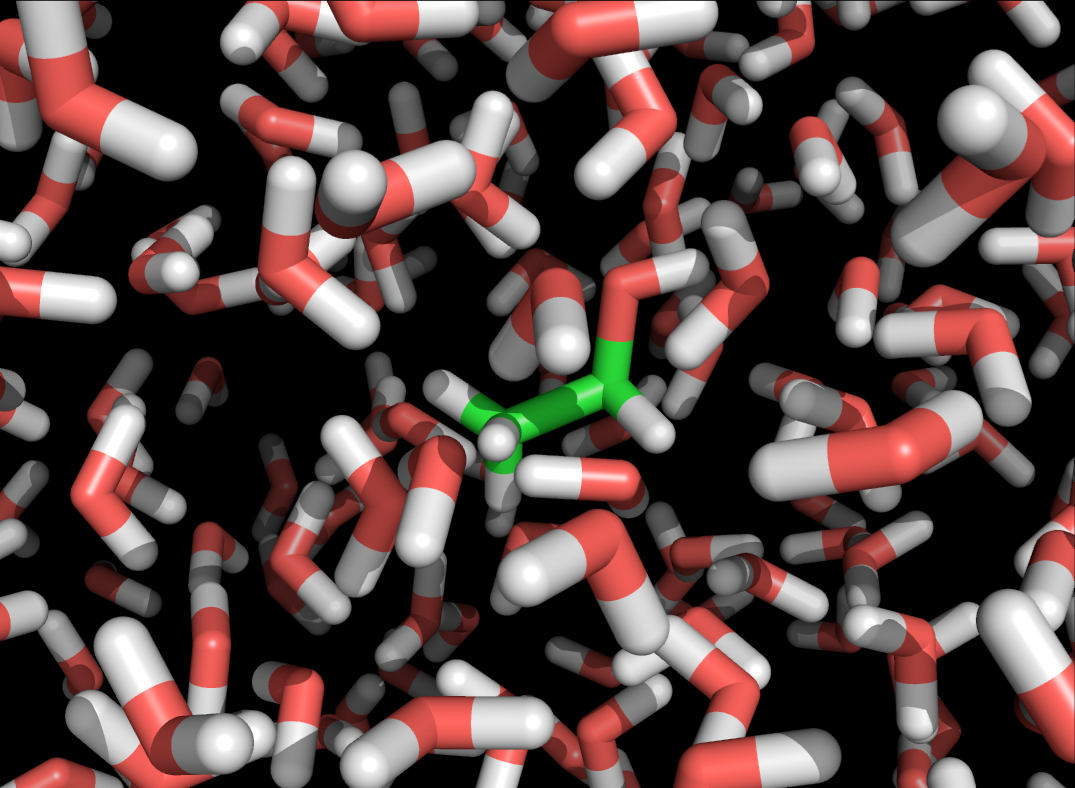Author:   Christian Blau, Joe Jordan, Erik Lindahl, Alessandra Villa and Artem Zhmurov
Goal:     Learn how to run a solvation free energy computations for a small solute using alchemical transformation and Bennett Acceptance Ratio (BAR) method
Prerequisites: Know how to run an MD simulation, basis knowledge of thermodynamics
Time:      90 minutes
Software:  GROMACS 2023 (and 2022)
Version:    1.0


## Background Change in free energy is a very important physical quantity that determines the direction of many biological processes. For instance, the solubility of a molecule in a solvent is determined by the solvation free energy - a change in free energy when taking a solute molecule from vacuum into the solvent. Binding free energy is very important when investigating the binding affinity of ligands (or drugs) to a specific binding site on the receptor. In this tutorial we will learn how to use one of the most popular approaches to evaluate difference in free energy of two states - alchemical free energy evaluation.

For simplicity, we will focus on the free energy of solvation for a small molecule (ethanol), but similar procedures are used to calculate binding free energy. We will start with some background on how the free energies can be calculated in molecular dynamics simulations, and how the evaluation of free energy of solvation is one of the steps, necessary to calculate free energy of binding. Then, we will focus on the practicalities of doing such a calculation in GROMACS.

# Calculating free energy¶

Assume that we need to compute the difference in free energy of two states $$A$$ and $$B$$. Let us also assume that $$A$$ and $$B$$ are well separated in the phase space and direct comparison between them is problematic. The trick then is to construct a set of intermediate states that are close to one another in the phase space. The proximity of the states allows one to compare them directly, computing the free energy difference between two consequent intemediate states. Then, going from one state to another, one can complete the full path between the states $$A$$ and $$B$$ and the sum of the differences between consequentive intermediate states will give the difference in free energies between $$A$$ and $$B$$.

For example, to calculate the binding free energy of the ligand to a receptor, we ultimately need to compare the state, where the ligand is bound to the receptor, to the state where both the ligand and the receptor are separated in solution:

This can be calculated directly, for example by slowly dragging the ligand away from the receptor and computing the dependence of average force between them. If the system will have enough time to explore all possible states while the ligand is pulled away, the integral of the force over the distance will give the free energy profile (usually called potential of mean force). However, the simulation times needed for convergence of this method are usually very long and when convergence is not reached, the fluctuations in forces can be very large. This makes the potential of mean force methods much more expensive than using free energy perturbation or alchemical free energy computation methods such as the Bennett Acceptance Ratio (BAR) that we’ll use in the tutorial.

Remember that a free energy difference between two states $$A$$ and $$B$$ ($$F_B - F_A$$) determines their relative probability $$p_A$$ and $$p_B$$,

$\frac{p_A}{p_B} = exp \frac{F_B - F_A}{k_BT}$

where $$k_B$$ is Boltzmann’s constant that relates thermal energy to the temperature ($$k_B = 1.38·10^{-23}$$ J/K), and $$T$$ is the temperature. We could, in principle, calculate a free energy difference by observing the system long enough, and measuring how often the system is in which state (i.e. measuring probabilities $$p_A$$ and $$p_B$$). The free energy differences, however, are often of the order of tens of kJ/mol. For example, the free energy of solvation of ethanol at 298K is -20.1 kJ/mol, which is equivalent to -8.1 $$k_BT$$ or to a relative probability of being in one of the states of $$3·10^{-4}$$. We would need to wait a long time for that transition to occur spontaneously, and even longer to get good statistics on it. To shorten the time needed for convergence, free energy methods rely on one basic idea: to force the system to where it doesn’t want to be, and then measure by how much it doesn’t want to be there. In potential of mean force, we apply mechanical force to decouple ligand and receptor. In free energy perturbation methods, we transform the system through a set of unphysical (alchemical) states, that connect two physical states we are interested in. To do so, the interaction strength between a molecule of interest and the rest of the system is coupled to a variable $$\lambda$$:

$E_{total} = E_{ligand-ligand} + E_{rest-rest} + \lambda E_{ligand-rest}$

and we slowly turn $$\lambda$$ from 1 to 0. This means we can effectively turn off a ligand, and pretend that it is no longer coupled to a system (at $$\lambda=0$$). This way we force the system to where it does not want to be (either to the solvated or to the vacuum state, depending on what the sign of the free energy difference is). We then use the Bennett Acceptance Ratio (BAR) method of calculating the free energy difference between these to states (which is a measure of “how much it does not want to be there”).

This method of coupling and de-coupling the receptor and ligand can help us with calculating the free energy of binding, because now we can create a two-step path: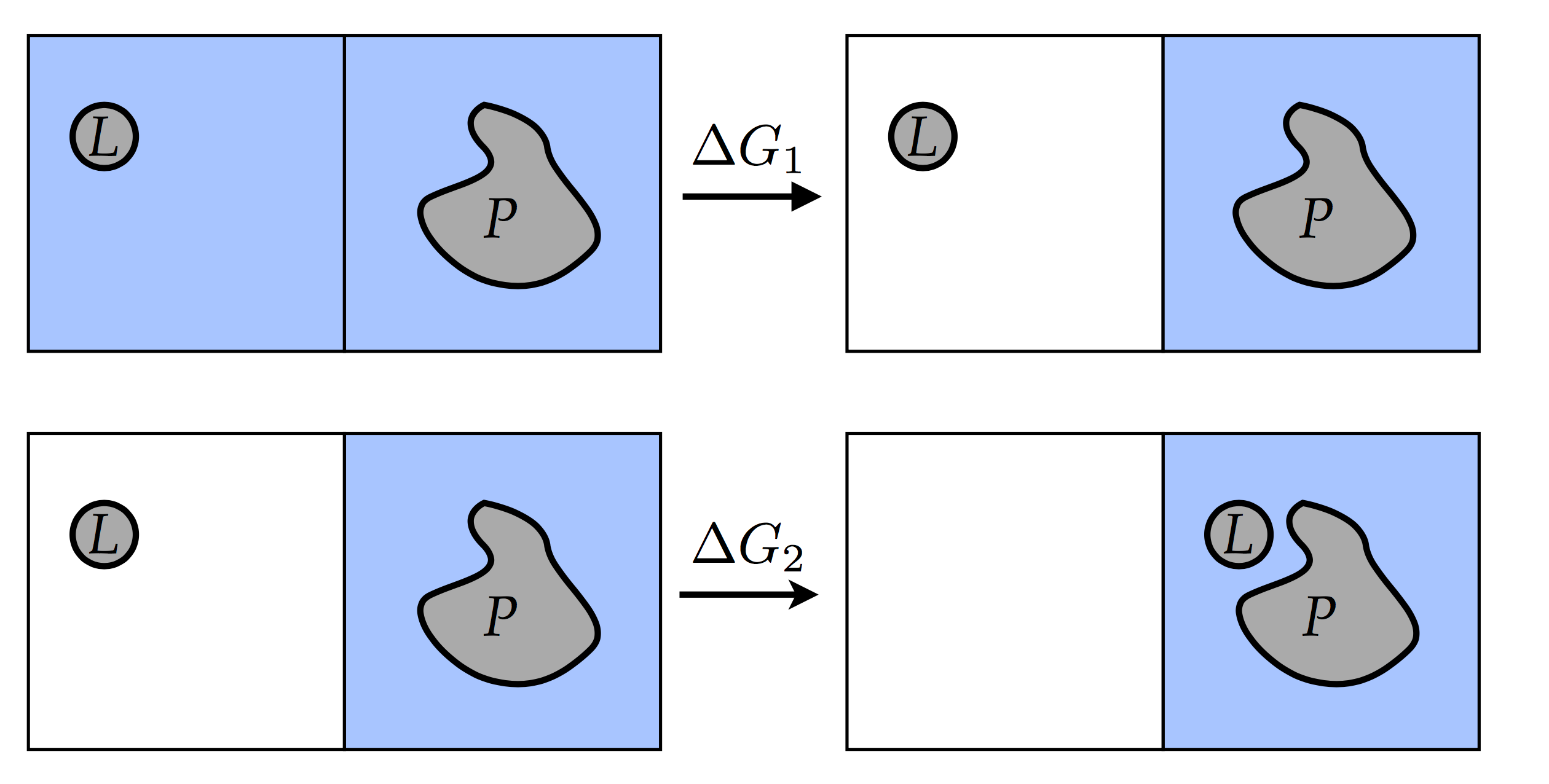where we first de-couple the ligand from the solvent (top panel), and then re-solvate the ligand in the presence of the receptor (bottom panel). The free energy of binding is thus

$\Delta G_{binding} = \Delta G_1 + \Delta G_2$

and the simulation is split into two parts: one is calculating the de-solvation free energy, and the other is calculating the free energy of introducing the ligand into the binding site on the receptor. The first simulation is the inverse of a free energy of solvation. That last simulation couples the ligand from $$\lambda=0$$ where it doesn’t interact with the system, to the state with $$\lambda=1$$, where the ligand is bound to the receptor. In this tutorial we will perform only the first step of calculating the solvation energy. Partly for computational performance reasons: because there is no protein involved, the simulation box size can be small and the simulations will be fast.

## Free energy of solvation

To calculate a free energy of solvation, we calculate $$-\Delta G_1$$ in the picture above, or, equivalently, $$\Delta G_{solv}$$ in this picture: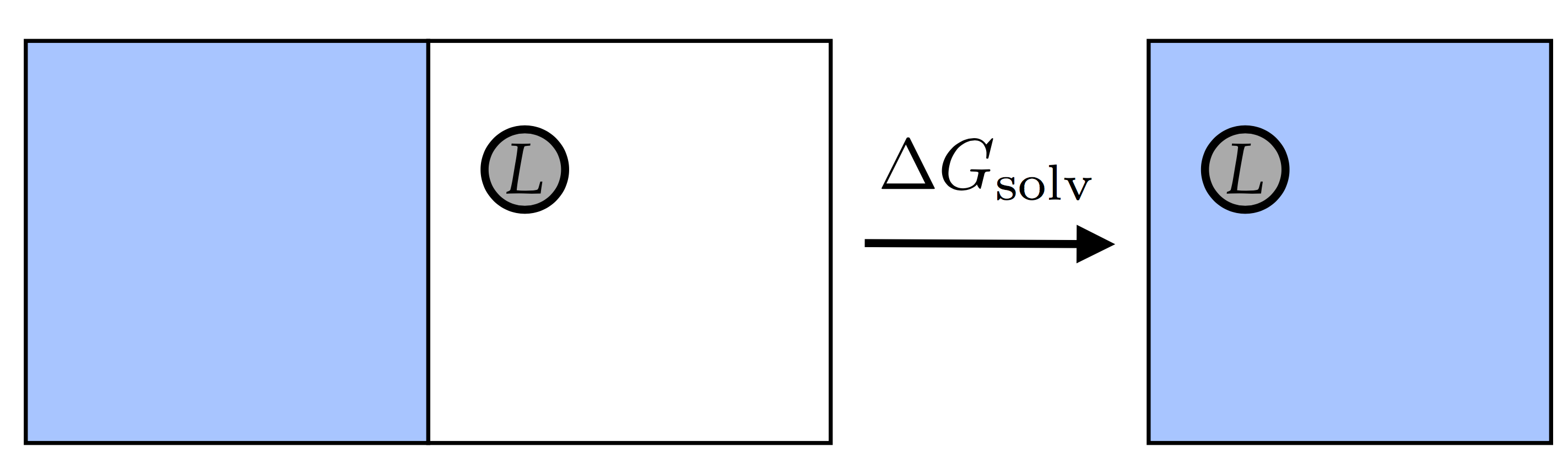We’ll do this by transforming the system through a set of unphysical (alchemical) step, that connect two physical states and use Bennett Acceptance Ratio (BAR) calculations using GROMACS (see GROMACS Manual for details).

The BAR method relies on computing the difference between two close states and hence requires the output of two simulations, say at states $$\lambda_A$$ and $$\lambda_B$$. The free energy difference can be calculated directly if $$\lambda_A$$ and $$\lambda_B$$ are close enough (see Bennett’s original article: Bennett, J. Comp. Phys, (1976) vol. 22 p. 245 for details), by calculating the Monte Carlo acceptance rates of transitions from $$\lambda_A$$ to $$\lambda_B$$ and vice versa, mapping states from $$\lambda_A$$ and $$\lambda_B$$. The term ‘close enough’ here means that switching between the two states should be possible in both directions and some of the configurations of the system should be allowed in both states (i.e. the states should share some parts of phase space).

The most obvious points for $$\lambda_A$$ and $$\lambda_B$$ would be $$\lambda_A=0$$ and $$\lambda_B=1$$. These end states, however, usually have very few system conformations in common: they share very little phase space. Because of this, we will never see enough transitions from one state to the other and the free energy computations will never converge in this case. That is why the transition is usually split into several intermediate unphysical states: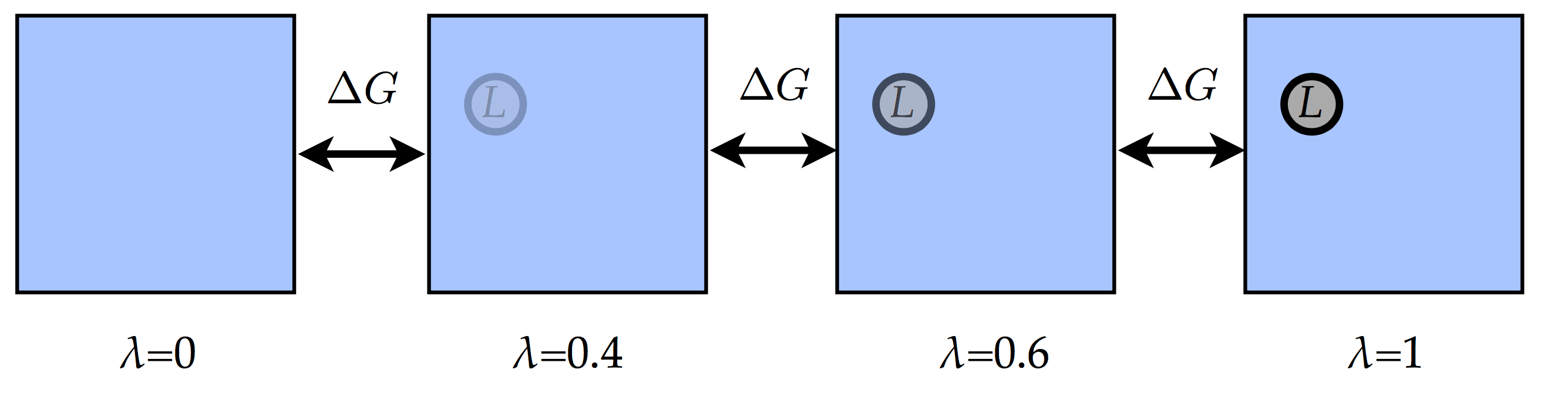with as many $$\lambda$$ points as are needed for the convergence between two nearby states (i.e. values of $$\lambda$$). We will therefore effectively ‘slowly’ turn on (or off) the interactions between the solute and the solvent. Then we will process the data from all these simulations, comparing the nearby values of $$\lambda$$. This means that we need to run as many simulations as there are $$\lambda$$ values and the data from simulation at $$\lambda=0.4$$, will be used to calculate the difference in free energy of states $$\lambda=0.2$$ and $$\lambda=0.4$$ and states $$\lambda=0.4$$ and $$\lambda=0.6$$.

Here we will use 7 $$\lambda$$ points: 0, 0.2, 0.4, 0.6, 0.8, 0.9 and 1. Combining the results of all the $$\lambda$$-point simulations will give us the total difference in free energy (i.e. the difference between the states with $$\lambda_A=0$$ and $$\lambda_B=1$$).

Compare to the state-of-the-art way of doing the free energy perturbation simulations, we will take one shortcut in this tutorial. We will turn off both the electrostatic (Coulomb) interactions and the Van der Waals (Lennard-Jones) interactions at the same time. For high-quality results, these stages are normally separated, but here we will do both of them at the same time for expediency. GROMACS uses ‘soft-core’ interactions to make sure that while the normal (Lennard-Jones and Coulomb) interactions are being turned off, there will never be two point charges sitting on top of each other: this is achieved by turning on an interaction that effectively repels particles at intermediate $$\lambda$$ points (in such a way that it cancels out from the free energy difference). For details on the soft-core interactions see here.

## Preparing the system Look for a file named topol.top, and a very basic coordinate file named ethanol.gro in the input files folder. This topology uses the OPLS force field and defines an ethanol molecule, and includes the definitions for SPC/E water, that will be used for solvation.

!cat input_files/topol.top

!cat input_files/ethanol.gro


Question: Take a look at the topology file topol.top. For the ethanol molecule definition, can you find which atoms are there, and how they are connected? Note, that we use standard OPLS atom types for the atoms in ethanol. These parameters were borrowed from the threonine side-chain, which has the same structure as the ethanol molecule. For more complex organic molecules one will have to look or to generate appropriate topology and coordinate files.

Before we start preparing the system, let us introduce a small piece of code that will allow us to interact with the command shell and filesystem:

import os,shutil

#simple function to write mdp files
def write_mdp(mdp_string, mdp_name, directory):
mdp_filename=os.path.join(directory,mdp_name)
mdp_filehandle=open(mdp_filename,'w')
mdp_filehandle.write(mdp_string)
mdp_filehandle.close()

#get the path to the working directory
pwd=os.getcwd()

#path to gromacs files
input_files=os.path.join(pwd,'input_files')
output_files=os.path.join(pwd,'output_files')
if not os.path.isdir(output_files):
os.mkdir(output_files)
shutil.copy(os.path.join(input_files,'ethanol.gro'),os.path.join(output_files,'ethanol.gro'))
shutil.copy(os.path.join(input_files,'topol.top'),os.path.join(output_files,'topol.top'))
%cd output_files


The write_mdp(…) function, defined here will be used to create mdp configuration files directly from this notebook. With all input files copied to the working directory, we can safely start working. Note, that you can always reset this tutorial by deleting the ‘output_files’ folder and executing the box above. Below, all the lines starting with ! indicate the shell commands.

First, we need to define the simulation box, which later will be filled with water solvent. The box should fully incorporate the solute with some space to spare:

!gmx editconf -f ethanol.gro -o box.gro -bt dodecahedron -d 1.2


This sets up the simulation box as a rhombic dodecahedron with a minimum distance between the solute (the ethanol molecule) and the box edge of 1.2 nm. The rhombic dodecahedron shape of the box provides more effective packing of periodic images in comparison to rectangular boxes and hence, fewer water molecules are needed to separate the periodic images of the ethanol molecule. See the [GROMACS manual] (http://manual.gromacs.org/current/reference-manual/algorithms/periodic-boundary-conditions.html) for illustrations of this box shape and how its periodic images are arranged.

The gmx editconf command defines the box, without adding the solvent to it. The later is done by the gmx solvate command below.

!gmx solvate -cp box.gro -cs -o solvated.gro -p topol.top


This will fill the box we created on the previous step with water molecules (roughly 500 of them). The solute will be taken from the file listed in the -cp option. The -cs option can be used to specify the template system that contais solvent molecules only. Leaving it blank will make GROMACS use the default solvent layout. The command will add the coordinates for the water molecules to the end of the gro file and will update the topology. You are strongly encouraged to investigate corresponding files.

The initial placement of water molecules is not ideal and may contain atoms that are too close to one another or small patches of empty spaces. The coordinates of the solute atoms may come from the experiment, where the placement of atoms is not precise. These, very small discrepancies in the atom positioning can carry a very substantial excess of potential energy and, as a result, the system will have very high interatomic forces. So high that the numerical integration of equation of motion will be unstable even for a very short timesteps. To overcome this and make the system configuration suitable for simulation, we will first perform the energy minimization. Executing the following cell will create the mdp file with the instructions to GROMACS to perform the energy minimization. The file will then be preprocessed by grompp into a em.tpr run file - the file that contains all the data necessary to perform the minimization, including coordinates of the atoms, topology and simulation protocol. Running the mdrun on the last line will execute the energy mimimization simulations.

em_mdp=""";minimal mdp options for energy minimization
integrator               = steep
nsteps                   = 500
coulombtype              = pme
"""
write_mdp(em_mdp,'em.mdp',output_files)
!gmx grompp -f em.mdp -c solvated.gro -o em.tpr
!gmx mdrun -v -deffnm em


## Equilibration of energy We are now ready to perform energy equilibration, a step necessary to achieve thermal equilibrium and uniformly distributh the energy over the system. For this step we will turn on pressure and temperature coupling: we are about to compute the difference in Gibbs free energy, and for that, the system must maintain both constant temperature and pressure, while the ethanol molecule is being de-coupled. For that, we will be using the velocity rescale thermostat, and the C-rescale barostat (here more on thermostat and barostat). The global equilibration is done with equil.mdp config file, constructed below. As with the mimization, the tpr file is then constructed and passed to GROMACS to run simulations.

equil_mdp=""";equilibration mdp options
integrator               = md
nsteps                   = 100000
dt                       = 0.002
nstenergy                = 100
rlist                    = 1.1
nstlist                  = 10
rvdw                     = 1.1
coulombtype              = pme
rcoulomb                 = 1.1
fourierspacing           = 0.13
constraints              = h-bonds
tcoupl                   = v-rescale
tc-grps                  = system
tau-t                    = 0.5
ref-t                    = 300
pcoupl                   = C-rescale
ref-p                    = 1
compressibility          = 4.5e-5
tau-p                    = 1
gen-vel                  = yes
gen-temp                 = 300
"""
write_mdp(equil_mdp,'equil.mdp',output_files)
!gmx grompp -f equil.mdp -c em.gro -o equil.tpr
!gmx mdrun -deffnm equil


Here you can use the mdrun option -ntmpi 1 to specify only using one mpi rank since the system is so small. For larger systems more mpi ranks would be appropriate. For very small systems such as this one, it would also be possible to use -nt 1 to specify only using one processor. You may want to adjust the number of cores used, but given the small system size, even one core should be sufficient to complete the run in about a minute. After this step we should be ready with a hopefully equilibrated configuration of ethanol in water, saved in equil.gro.

Question: You should check whether the system has been equilibrated. How could you do this?

Note: if you do not want to wait, but look at some of the results directly, copy the data from the /reference directory into the output_files directory. To do this from within this notebook, remove the comment characters (#) in the following cell

## ONLY execute the lines below if you do not want to run and wait for the simulations to finish.
#Before executing the command below, check that you are in output_files directory
#!cp -r ../reference/equil.* ./


# Creating the $$\lambda$$ points¶

Now the system preparation is complete and we are ready to do a production simulations. First, we need to define different $$\lambda$$ points, each of which indicate the alchemical state along the transition between solvated and in vacuo states. We define those states in the mdp configuration file below with consecutive values of $$\lambda$$. The free energy settings state the following: take the molecule ethanol, and couple it to our variable $$\lambda$$. This is done so that $$\lambda=0$$ means that the molecule is de-coupled, and $$\lambda=1$$ means that the molecule is fully coupled. Take a look at the couple-lambda0 and couple-lambda1 options in the file. There, vdwq means that both Lennard Jones and Coulomb will be affected by the value of $$\lambda$$, hence the solute molecule will be fully decoupled. What is decoupled is be selected by the couple-moltype option (ethanol in our case). The sc-power, sc-sigma and sc-alpha settings control the ‘soft-core’ interactions that prevent the system from having overlapping particles as it is being de-coupled. In this tutorial we use Beutler’s softcore developed by Beutler and coworkers.

A full description of the simulation parameters for free energy calculation in GROMACS can be found here.

run_mdp="""; we'll use the sd integrator (an accurate and efficient leap-frog stochastic dynamics integrator) with 100000 time steps (200ps)
integrator               = sd
nsteps                   = 100000
dt                       = 0.002
nstenergy                = 1000
nstcalcenergy            = 50 ; should be a divisor of nstdhdl
nstlog                   = 5000
; cut-offs at 1.0nm
rlist                    = 1.1
rvdw                     = 1.1
; Coulomb interactions
coulombtype              = pme
rcoulomb                 = 1.1
fourierspacing           = 0.13
; Constraints
constraints              = h-bonds
; set temperature to 300K
tc-grps                  = system
tau-t                    = 2.0
ref-t                    = 300
; set pressure to 1 bar with a thermostat that gives a correct
; thermodynamic ensemble
pcoupl                   = C-rescale
ref-p                    = 1.0
compressibility          = 4.5e-5
tau-p                    = 5.0

; and set the free energy parameters
free-energy              = yes
couple-moltype           = ethanol
nstdhdl                  = 50 ; frequency for writing energy difference in dhdl.xvg, 0 means no ouput, should be a multiple of nstcalcenergy.
; these 'soft-core' parameters make sure we never get overlapping
; charges as lambda goes to 0
; soft-core function
sc-power                 = 1
sc-sigma                 = 0.3
sc-alpha                 = 1.0
; we still want the molecule to interact with itself at lambda=0
couple-intramol          = no
couple-lambda1           = vdwq
couple-lambda0           = none
init-lambda-state        = {}
; These are the lambda states at which we simulate
; for separate LJ and Coulomb decoupling, use
fep-lambdas              = 0.0 0.2 0.4 0.6 0.8 0.9 1.0
"""


The grompp and mdrun are executed sequentially for each $$\lambda$$ point. This can be optimized by using the -multirun option or executing the commands in parallel, but it is not necessary for the system of our size. You may want to use more than one core though.

number_of_lambdas=7
for lambda_number in range(number_of_lambdas):
lambda_directory=os.path.join(output_files,'lambda_{:0>2}'.format(lambda_number))
os.mkdir(lambda_directory)
gro_file=os.path.join(output_files,'equil.gro')
top_file=os.path.join(output_files,'topol.top')
shutil.copy(gro_file,os.path.join(lambda_directory,'conf.gro'))
shutil.copy(top_file,lambda_directory)
write_mdp(run_mdp.format(lambda_number),'grompp.mdp',lambda_directory)
%cd $lambda_directory !gmx grompp !gmx mdrun  The script has created a directory for each $$\lambda$$ point containing the corresponding simulation parameter files. For example directory lambda_00 contains a grompp.mdp file with option init-lambda-state = 0 (that corresponds to $$\lambda$$=0), directory lambda_01 a grompp.mdp file with option init-lambda-state = 1 (that corresponds to $$\lambda$$=0.2). %cd ..  !ls -F  !cat lambda_00/grompp.mdp  Note: if you do not want to wait, but look at some of the results directly, copy the data from the /reference directory into the output_files directory. To do this from within this notebook, remove the comment characters (#) in the following cell ## ONLY execute the lines below if you do not want to run and wait for the simulations to finish. Before executing the command below, check that you are in output_files directory #!cp -r ../reference/lambda_00 ./ #!cp -r ../reference/lambda_01 ./ #!cp -r ../reference/lambda_02 ./ #!cp -r ../reference/lambda_03 ./ #!cp -r ../reference/lambda_04 ./ #!cp -r ../reference/lambda_05 ./ #!cp -r ../reference/lambda_06 ./  ## Post-processing: extracting the free energy After the simulations are done, we can extract the full free energy difference from the output data. Check your directories lambda_00 to lambda_06 for files called dhdl.xvg. These contain the energy differences that are going to be used to calculate the free energy difference.: !head -40 lambda_00/dhdl.xvg  Now we combine the dhdl.xvg files into a free energy with the GROMACS BAR tool gmx bar: bar_string = '' for lambda_number in range(number_of_lambdas): lambda_directory=os.path.join(output_files,'lambda_{:0>2}'.format(lambda_number)) bar_string=bar_string + lambda_directory + '/dhdl.xvg ' !gmx bar -b 100 -f$bar_string


Where the -b 100 means that the first 100 ps should be disregarded: they serve as an extra equilibration step, this time with the $$\lambda$$ value already set. An alternative to the script is to run the command line ‘gmx bar -b 100 -f lamdba_0?/dhdh.xvg’ from the output_files directory

Look for the final number in the output, it should be close to the experimental value. With appropriate sampling you should get a value of approximately -19.1 +/- 0.3 kJ/mol (see for example J. Phys. Chem. B 2006). As part of the free energy code in mdrun, we have already calculated the offset enthalpies to the adjacent $$\lambda$$ points, so this is already available in the dhdl.xvg file (together with all information about the point itself, what simulation it was, etc) - no need to rerun any simulations or store the entire trajectories. The gmx bar command will do all the complicated processing needed for Bennett Acceptance Ratio free energies and just give you the results.

Question: Longer runs will change the free energy value a bit as the standard error estimate shrinks (try it). Why can there sometimes be a significant (i.e. bigger than the estimated error) difference between the experimental result and the simulation result? How could this be improved?

Question: Look at the error bars for the individual $$\lambda$$ points: they vary a lot between individual point pairs. What does this mean for the efficiency for the overall calculation? How could it be improved?

## Relative free energy of solvation Here we show how to set-up the system to calculate relative free energy. As example we take the alchemical transformation from ethanol to ethanethiol in water solution. First we look at the topology file that describes the transformation from ethanol (state A) to ethanethiol (state B):

#Before executing the command below, check that you are in output_files directory
!cat ../input_files/ethanol_Sethane.top


For the safe of time, we use an already solvated and equilibrated system. To solvate and equilibrate the system we have use the procedure reported at the begin of the tutorial.

## ONLY execute the lines to copy in output_files directory the gro and top files after equilibration
#!cp ../reference/relative_solvation/ethanol_Sethane.gro ./
#!cp ../reference/relative_solvation/ethanol_Sethane.top ./


Here you find the mdp parameter to perform the alchemical transformation

run_rel_mdp="""; we'll use the sd integrator (an accurate and efficient leap-frog stochastic dynamics integrator) with 100000 time steps (200ps)
integrator               = sd
nsteps                   = 100000
dt                       = 0.002
nstenergy                = 1000
nstcalcenergy            = 50 ; should be a divisor of nstdhdl
nstlog                   = 5000
; cut-offs at 1.0nm
rlist                    = 1.1
rvdw                     = 1.1
; Coulomb interactions
coulombtype              = pme
rcoulomb                 = 1.1
fourierspacing           = 0.13
; Constraints
constraints              = h-bonds
; set temperature to 300K
tc-grps                  = system
tau-t                    = 2.0
ref-t                    = 300
; set pressure to 1 bar with a thermostat that gives a correct
; thermodynamic ensemble
pcoupl                   = C-rescale
ref-p                    = 1.0
compressibility          = 4.5e-5
tau-p                    = 5.0

; and set the free energy parameters
free-energy              = yes
nstdhdl                  = 50 ; frequency for writing energy difference in dhdl.xvg, 0 means no ouput, should be a multiple of nstcalcenergy.
; these 'soft-core' parameters make sure we never get overlapping
; charges as lambda goes to 0
; soft-core function
sc-power                 = 1
sc-sigma                 = 0.3
sc-alpha                 = 1.0
; we still want the molecule to interact with itself at lambda=0
init-lambda-state        = {}
; These are the lambda states at which we simulate
; for separate LJ and Coulomb decoupling, use
fep-lambdas              = 0.0 0.2 0.4 0.6 0.8 0.9 1.0
"""

Question: In what differs the mdp parameter file above with the one use to calculate the free energy of solvation?
Exercise: Following the procedure above, calculate the relative free energy for the transformation from ethanol to ethanethiol in water solution. Based on your results which molecule is more hydrophylic?
Tips: use different paths or different input/output names for each type of calculations.

# Where to go from here¶

After calculating the free energy of solvation, we’ve solved the first part of the free energy of binding of the earlier equations. The second part involves coupling a molecule into (or out of) a situation where it is bound to a protein. This introduces one additional complexity: we end up with a situation where a weakly coupled ligand wanders through our system: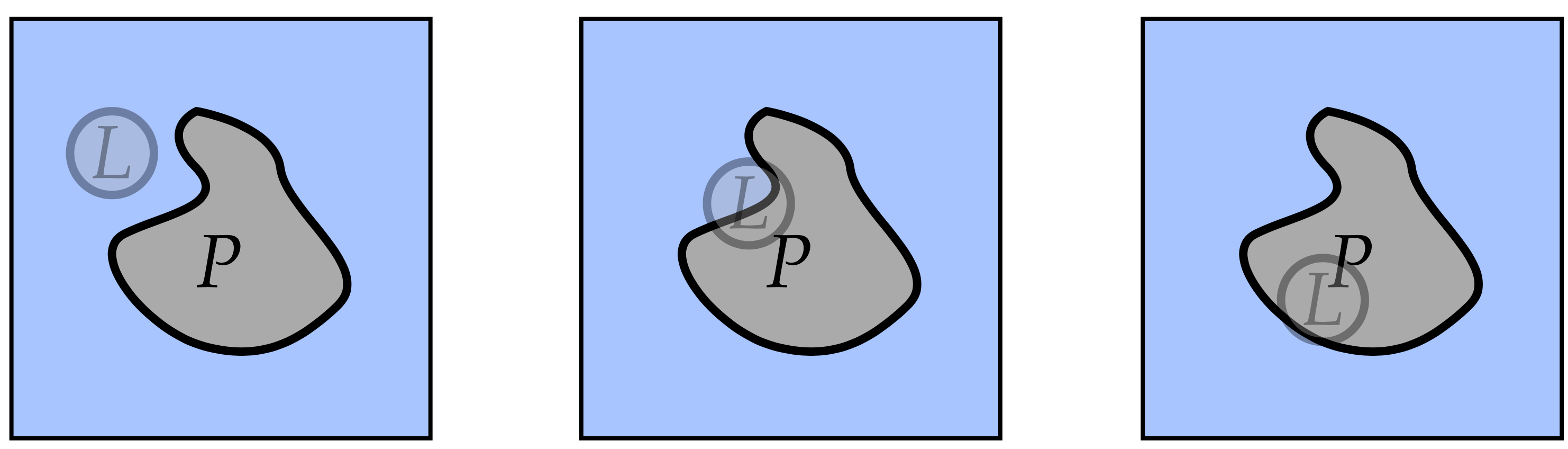which is bad because this is a poorly reversible situation: there are suddenly very few states that map from a weakly coupled to a more strongly coupled molecule, which will drastically reduce the accuracy of the free energy calculation.

This situation can be remedied by forcing the ligand to stay at a specific position relative to the protein. This can be done with the GROMACS ‘pull code’, which allows the specification of arbitrary forces or constraints onto with respect to centers of mass of any chosen set of atoms onto any other group of atoms. With a pull type of ‘umbrella’, we can specify that we want a quadratic potential to this specified location, forcing the ligand to stay at its native position even when it has been fully de-coupled.

One way find out where to put the center of the force is by choosing a group of atoms in the protein close to the ligand, and doing a simulation with full ligand coupling, where the pull code is enabled, but with zero force. The pull code will then frequently output the coordinates of the ligand, from which an average position and an expected deviation can be calculated. This can then serve as a reference point for the location of the center of force for the pull code during the production runs, and the force constant of the pull code.

Once the free energy has been calculated, care must be taken to correct for the fact that we have trapped our molecule. This can easily be done analytically.

Optional Question: Given a measured standard deviation in the location of the center of mass of our ligand, how do we choose the force constant for the pull code?

Optional Question: How do we correct for using the pull code: what is the contribution to the free energy of applying a quadratic potential to a molecule?